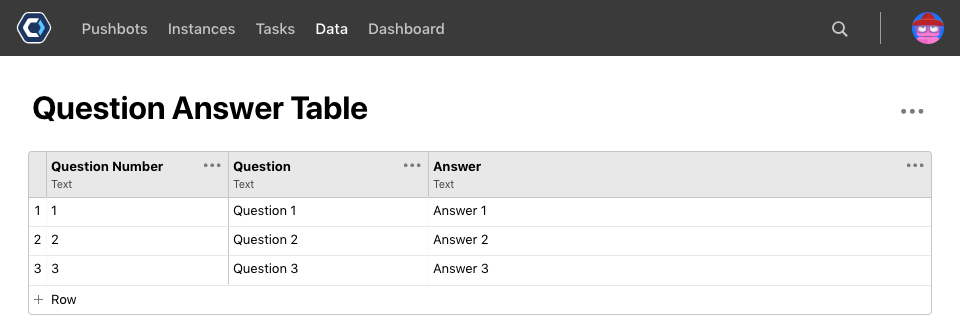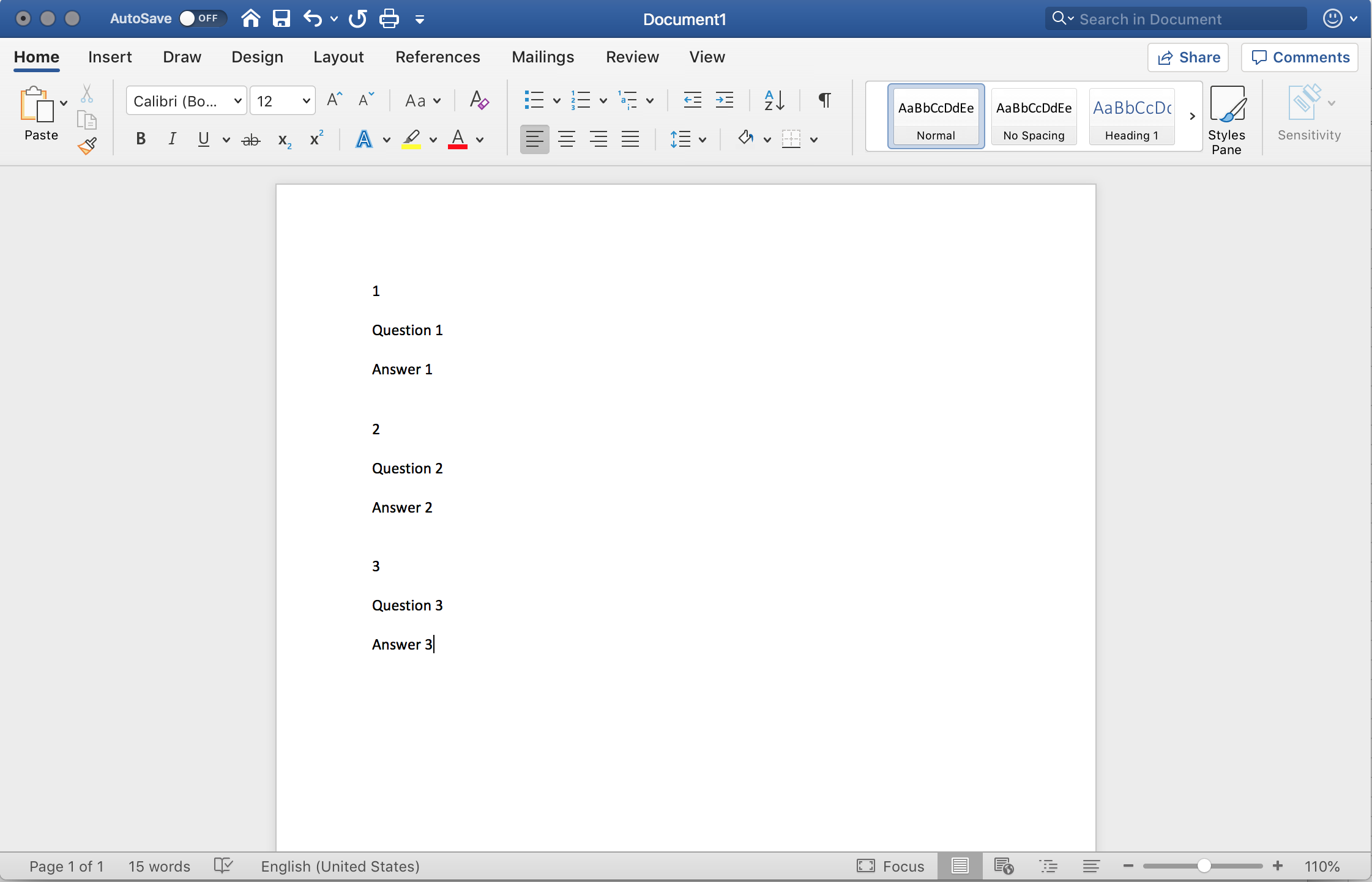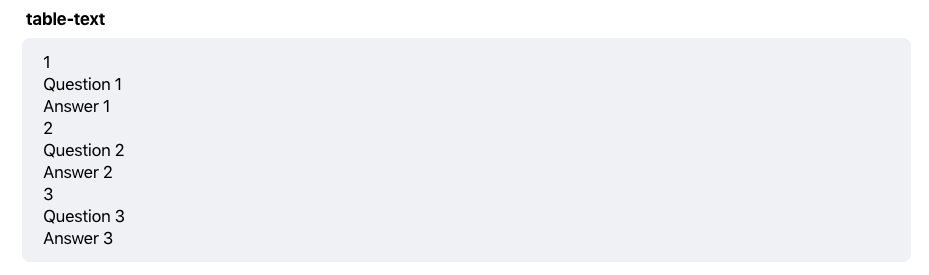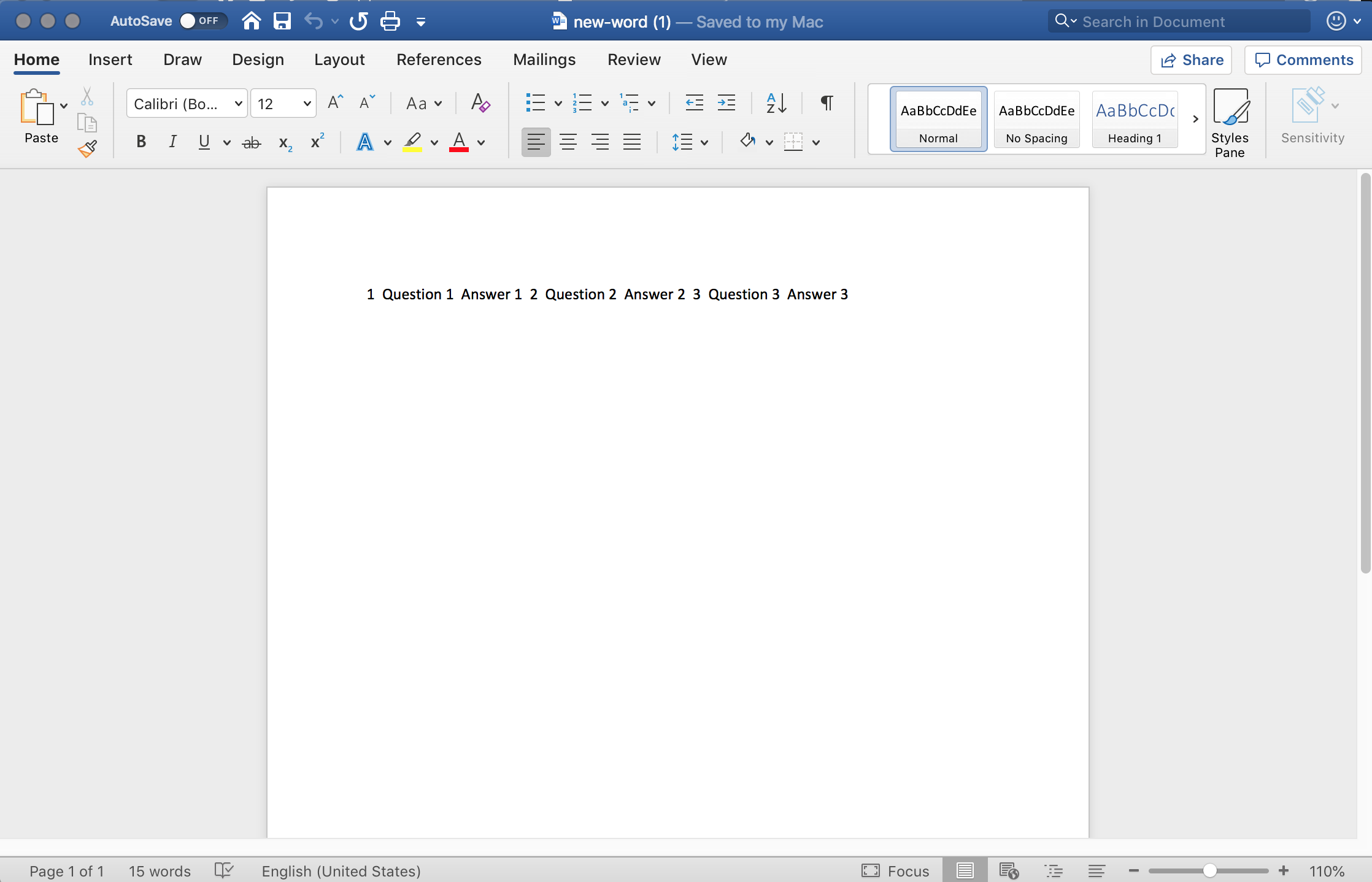# Summarize all csv cells into fields

My goal is to place the contents of a data table or CSV file into a Word and PowerPoint template.

I have an example table and example output Word document below. My table includes one question and one answer per row. CSV: Summarize rows with formulas outputs as many fields as you need and I need a field for each cell.

Can I use CSV: Summarize rows with formulas to do the following (?):

• Turn every row into an array (ex: `{"1", "Question 1", "Answer 1"}`)
• For every row array, turn every list item into a field reference (ex: Question Number Row 1, Question Row 1, Answer Row 2)
• Repeat based on the number of rows

Open to alternative approaches.Tagged:

• Could you just use Tables: Convert data table to text instead of the row formulas?
Set the row template as:

columns['Question Number']
columns['Question']

And then set the row delimiter to \n\n or \n to space it out.
That should give the output you're showing as text. From there I'd think you can put it into a word doc or anything easily. Unless you need each data point for a field, in which case I would think you could solve the issue the way you're describing.

• Thanks @Kevin_949344!

I received the table as one field (image below). I will need a field for each value, though, since the cell in each row has a distinct placement in the PowerPoint file. (One question answer per slide)

Also, Word: Create a Word Document does not preserve line break formatting for the field. I could try Text: Find text next to other text to save fields by line, but the initial approach may be more efficient since the row count can differ.